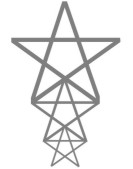All about flooble | fun stuff | Get a free chatterbox | Free JavaScript | Avatarsperplexus dot infoStar Stack (Posted on 2004-04-12)Three pentagram-shaped stars (the stars formed from the diagonals of a regular pentagon) are stacked up so that the bottom two ends of the tips touch the middle ends of the tips of the star below. (See diagram.)

The distance from the top of the stack to the floor (where the bottom star's "feet" rest) is 4 feet.

What is the distance between the bottom two ends of the tips of the stack that touch the floor?

 No Solution Yet Submitted by Gamer Rating: 3.5000 (6 votes)Comments: ( Back to comment list | You must be logged in to post comments.)solution| Comment 1 of 19

Taking the perpendicular distance from the top point to the horizontal bar on the top star to be 1 unit, the edge of one arm of the top star is 1/sin72, and half the edge of the pentagon in the largest circle is 1/tan72.  Twice the sum of these numbers is the length of one full straight line on the star.

Further trigonometry yields the ratio of the sides of the smaller star to those of the larger star as 1/phi where phi is the golden ratio.  The distance between the leg points of the largest star is 2 cos 36 / sin 72 in the arbitrary units we are using.

The total height of the top star is (2/sin72 + 2/tan72) / sin 72.

The "bottom part" (i.e., excluding the top ray) of the top star is then just this value minus 1.

The height of the whole set of 3 is then the height of the top star plus the bottom parts of the smaller stars, which are respectively 1/phi and 1/phi^2 times that of the bottom part of the top star. This totals, to the accuracy shown, 4.788854381999831, so the unit being used is 4/4.788854381999831 of a foot.

The bottom feet points are separated by the distance of the top feet points divided by phi^2.  The distance between the bottom two feet points comes out, converted to feet, as .5427931948888689 feet, if I did my arithmetic right.

 Posted by Charlie on 2004-04-12 12:17:31Please log in:

 Search: Search body:
Forums (2)